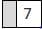Pandu and Raghu wrote two 2-digit numbers, ea...

### Related TestPandu and Raghu wrote two 2-digit numbers, each having the digit 7 only once and in the place shown.
PanduRaghu
If Pandu wrote the greatest two digit number and Raghu wrote the least two digit number, what is their product?
• a)
990
• b)
6790
• c)
6887
• d)
7663PRAVIN MANIKThe greatest two digit number written by Pandu is 97. The least two digit number written by Raghu is 70. Their product = 97 × 70 = 6790

 1 Crore+ students have signed up on EduRev. Have you?Mehala Selvam
Pandu and Raghu wrote two 2-digit numbers, each having the digit 7 only once and in the place shown.PanduRaghuIf Pandu wrote the greatest two digit number and Raghu wrote the least two digit number, what is their product?a)990b)6790c)6887d)7663Correct answer is option 'B'. Can you explain this answer?
The greatest two digit number written by pandu is 97 and by raghu is 70 now we want to see the product we do these two numbers multiply we get the answer as b (6790)View courses related to this question Explore Class 4 courses
 Explore Class 4 coursesView courses related to this question• ### The product of two numbers is 476. If one number is 34, what is the other n... more(Scan QR code)